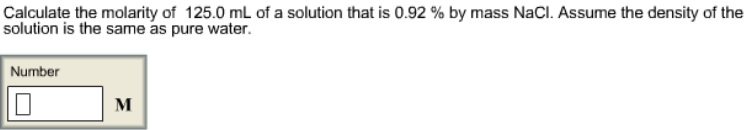# Problem: Calculate the molarity of 125.0 mL of a solution that is 0.92 % by mass NaCl. Assume the density of the solution is the same as pure water.

###### FREE Expert Solution
79% (54 ratings)###### Problem Details

Calculate the molarity of 125.0 mL of a solution that is 0.92 % by mass NaCl. Assume the density of the solution is the same as pure water.